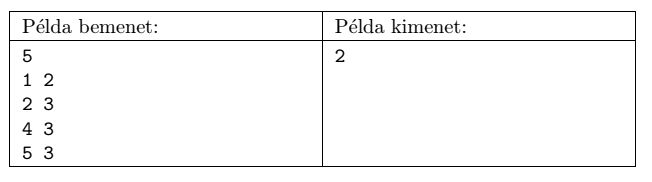Mathematical and Physical Journal
for High Schools
Issued by the MATFUND Foundation
 Already signed up? New to KöMaL?

#Problem S. 90. (May 2014)

S. 90. A cave consists of some chambers and passages connecting the chambers. A secret agent hid a valuable gem in one of the chambers. We would like to recover this treasure. The following information is known about the cave: it has $\displaystyle 1\le N\le 200\;000$ chambers, and there is exactly one path between any two chambers. We would like to find the gem without entering the cave by asking questions from the secret agent about the gem's location. The form of a question is always Is the gem located in the $\displaystyle i^\mathrm{th}$ chamber?'' The agent answers this question, and if the answer is No'', then also tells us the proper direction to find the gem. The difficulty lies in the fact that the agent wants to avoid any unnecessary questioning. So your task is to minimize the number of questions you ask from the agent. The maximal number of points for this exercise can only be obtained if your program correctly determines the minimal number of questions to locate the gem even if one should ask the maximal possible number of questions. In other words, your goal is to give the minimum number of questions that guarantee the recovery of the gem.

Your program should read the value of $\displaystyle N$ from the first line of the standard input, then the next $\displaystyle N-1$ lines containing the map of the cave: each line contains a pair of integers $\displaystyle a_i$, $\displaystyle b_i$ representing a direct passage between chambers $\displaystyle a_i$ and $\displaystyle b_i$. Your solution, the minimal number of questions, should be written to the first and only line of the standard output. In the example, Példa bemenet'' and Példa kimenet'' are a sample input and the corresponding output.Scoring and bounds. You can obtain 1 point for a brief and proper documentation clearly describing your solution. Nine further points can be obtained provided that your program solves any arbitrary valid input within 1 second of running time. We will run your program on several test cases. We will count it as a critical error if your program produces an output that is less than the necessary minimum. However, you can get partial points if your output is more than the optimum. Recall that the recovery of the gem must be guaranteed by asking the appropriate number of questions. Partial points can be obtained if your program yields a solution

$\displaystyle \bullet$ for $\displaystyle N\le 200$, or

$\displaystyle \bullet$ for $\displaystyle N\le 5000$.

The source code (s90.pas, s90.cpp, ...) without the .exe or any other auxiliary files generated by the compiler but with a short documentation (s90.txt, s90.pdf, ...), also describing which developer environment to use for compiling the source, should be submitted in a compressed file s90.zip.

(10 pont)

Deadline expired on June 10, 2014.

### Statistics:

 9 students sent a solution. 10 points: Makk László. 7 points: 1 student. 6 points: 1 student. 5 points: 1 student. 3 points: 2 students. 2 points: 1 student. 1 point: 1 student. Unfair, not evaluated: 1 solutions.

Problems in Information Technology of KöMaL, May 2014